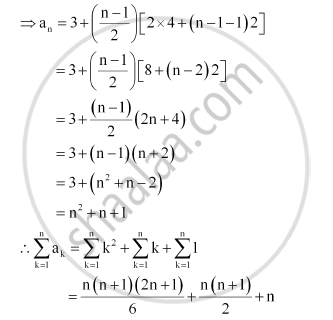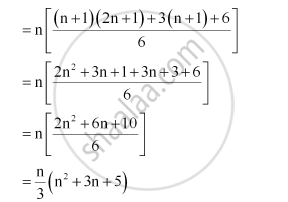CBSE (Arts) Class 11CBSE
Share
Notifications

View all notifications
Books Shortlist
Your shortlist is empty

# Find the Sum of the First N Terms of the Series: 3 + 7 + 13 + 21 + 31 + … - CBSE (Arts) Class 11 - Mathematics

Login
Create free account

Forgot password?

#### Question

Find the sum of the first n terms of the series: 3 + 7 + 13 + 21 + 31 + …

#### Solution

The given series is 3 + 7 + 13 + 21 + 31 + …

S = 3 + 7 + 13 + 21 + 31 + …+ an–1 an

S = 3 + 7 + 13 + 21 + …. + an – 2 a– 1 + an

On subtracting both the equations, we obtain

S – S = [3 + (7 + 13 + 21 + 31 + …+ an–1 an)] – [(3 + 7 + 13 + 21 + 31 + …+ an–1) an]

S – S = 3 + [(7 – 3) + (13 – 7) + (21 – 13) + … + (an – an–1)] – an

0 = 3 + [4 + 6 + 8 + … (n –1) terms] – an

an = 3 + [4 + 6 + 8 + … (n –1) terms]Is there an error in this question or solution?

#### APPEARS IN

NCERT Solution for Mathematics Textbook for Class 11 (2018 to Current)
Chapter 9: Sequences and Series
Q: 23 | Page no. 200
Solution Find the Sum of the First N Terms of the Series: 3 + 7 + 13 + 21 + 31 + … Concept: Concept of Series.
S